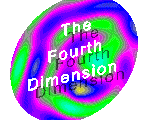Newton's Three Laws & Universal Gravitation

By Danny Keck

Few will argue that Sir Isaac Newton ranked among the top scientists of all time, and there are many that would go so far as to say that no one has had a more important effect on the development of science. Newton in fact did make some very important advances, such as creating infinitesimal calculus. In this paper I will briefly explain two other achievements of Newton: the laws of motion and universal gravitation.

Both ideas were published in Newton's most famous publication, Mathematical Principles of Natural Philosohy, printed in 1687. Newton first formulated his three laws of motion, which described the relationship between force and its result, acceleration. The first law states that a body will move at a constant speed unless a force acts upon it. Also an object at rest will stay at rest unless a force acts on it. If no force acts on an object that it will continue moving at a constant (or zero) speed.

This was originally thought up by Galileo, and it ran against conventional wisdom at that time. It was believed in the past that for an object to move at all a force must be acting on it; only an object at rest was not being affected by a force. Galilieo wondered however that if a perfectly smooth object were moving along a perfectly smooth horizontal surface, that it might travel forever in a straight line without any force to cause it to.

In his book Newton clearly stated that this would be true. Velocity is not the result of force, but rather acceleration is the result. This solved a problem that had been debated for centuries: what force could possilby be moving the planets? The answer is none; the planets are moving naturally in a uniform circular motion. Although gravity causes their direction to change constantly, their actual rate of movement is the same because there is no force to acclerate them.

The second law is a mathematical treatment of the first law. It lays down in simple yet accurate terms the numbers that desribe how a force changes the motion of a body. The acceleration of a mass is directly proportional to the force applied to it and inversely proportional to the mass of the object. All these reactions can be described with this simple formula: F=Ma. The second law can be considered the most important, as all of dyamics' basic equations can be derived from it using calculus.

If more than once force is acting on an object, than they must all be added together to compute the equation. The magnitude and direction of every force must be combined to form the resultant force, which is used in the equation. Acceleration is also a vector quantity and it is in the same direction as the force.

Newton's third law is popularly know as: for every action there is an equal and opposite reaction. It may be more accurate to say that the actions of two bodies on each other are always equal in magnitude and opposite in direction. If you press your hand against a wall with a certain amount of force, the wall will push back against your hand with the same amount of force. An airplane flying through the air is affected by the force of the earth pulling down on it, and in turn the airplane exerts an equal force on the earth (although because of the earth's immense mass this pull has a negligible effect.)

Legend has it that Newton was considering his Third Law one day when he saw an apple fall from a tree. He noted that the earth was pulling on the apple and the apple was pulling on the earth. He then wondered: what if the same force that was acting here also was responsible for keeping the whole universe together? What if earth and the sun attracted each other using the same concepts and formulas that gravity affects objects on earth?

It turned out that he was right. Earth's force of gravity is actually in effect throughout the entire universe, giving this force the name of universal gravitation. Its effects are very far reaching. Every pair of particles of matter in the universe attract each other with some gravitational force. The force acts in a straight line between them and is directly proportional to the combined masses of the objects and inversely proportional to the square of the distance separating them.

This can be calucated using this formula: F = (Gm1m2) / d2. G is the gravitational constant. It is a constant that is the same throughout the universe and never depends on anything. The value of G is approximately 6.67´10-11. This value is obviously very small but it still affects answers. Some scientists believe that the value of G is actually diminishing as time goes by, but very slowly.

The gravitational force is one of the four fundamental forces of the universe, along with the electromagnetic, strong nuclear and weak nuclear forces. Gravity is fairly weak. Between a proton and electron the force of gravity is 5´10-40 times as strong as the electromagnetic force. However, gravity does have an infinite range.

Although Newton's theories were landmark achievements and are usually very accurate, in some situations they fall apart. Einstein came up with the theory of relativity to fix some discrepencies in Newton's work, introducing ideas such as spacetime and gravity waves. The increased accuracy comes at a price; Einstein's formulas are far more complicated than Newton's.

The normal person working with normal objects, however, will find that Newton's theories work as accurately as can be measured. Newton's ideas on motion both on Earth and in the rest of the universe brought light to the confusing world of science, answered many of the great questions of the world, and were immense steps in the progression of mankind's ongoing scientific endeavors.